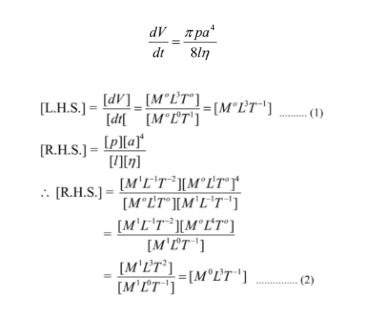# Dimensional Analysis

We quantify the size and shape of things using Dimensional Analysis. It helps us study the nature of objects mathematically. It involves lengths and angles as well as geometrical properties such as flatness and straightness. The basic concept of dimension is that we can add and subtract only those quantities that have the same dimensions. Similarly, two physical quantities are equal if they have the same dimensions.

## Dimensional Analysis Explained

The study of the relationship between physical quantities with the help of dimensions and units of measurement is termed as dimensional analysis. Dimensional analysis is essential because it keeps the units the same, helping us perform mathematical calculations smoothly.

### Unit Conversion and Dimensional Analysis

Dimensional analysis is also called Factor Label Method or Unit Factor Method because we use conversion factors to get the same units. To help you understand the stated better, let’s say you want to know how many meters make 3 km?

We know that 1000 meters make 1 km,

Therefore,

3 km = 3 × 1000 meters = 3000 meters

Here, the conversion factor is 1000 meters.

### Using Dimensional Analysis to Check the Correctness of Physical Equation

Let’s say that you don’t remember whether

1. time = speed/distance, or
2. time = distance/speed

We can check this by making sure the dimensions on each side of the equations match.

Reducing both the equations to its fundamental units on each side of the equation, we get

1. $$[T]=\frac{[L][T]^{-1}}{L}=[T]^{-1}$$ (Wrong)
2. $$[T]=\frac{[L]}{[L][T]^{-1}}=[T]$$ (Right)

However, it should be kept in mind that dimensional analysis cannot help you determine any dimensionless constants in the equation.

## Homogeneity Principle of Dimensional Analysis

Principle of Homogeneity states that dimensions of each of the terms of a dimensional equation on both sides should be the same. This principle is helpful because it helps us convert the units from one form to another. To better understand the principle, let us consider the following example:

Example 1: Check the correctness of physical equation s = ut + ½ at2. In the equation, s is the displacement, u is the initial velocity, v is the final velocity, a is the acceleration and t is the time in which change occurs.

Solution:

We know that L.H.S = s and R.H.S = ut + 1/2at2

The dimensional formula for the L.H.S can be written as s = [L1M0T0] ………..(1)

We know that R.H.S is ut + ½ at2 , simplifying we can write R.H.S as [u][t] + [a] [t]2

[L1M0T-1][L0M0T-1] +[L1M0T-2][L0M0T0]

=[L1M0T0]………..(2)

From (1) and (2), we have [L.H.S] = [R.H.S]

Hence, by the principle of homogeneity, the given equation is dimensionally correct.

### Applications of Dimensional Analysis

Dimensional analysis is a fundamental aspect of measurement and is applied in real-life physics. We make use of dimensional analysis for three prominent reasons:

• To check the consistency of a dimensional equation
• To derive the relation between physical quantities in physical phenomena
• To change units from one system to another

### Limitations of Dimensional Analysis

Some limitations of dimensional analysis are:

• It doesn’t give information about the dimensional constant.
• The formula containing trigonometric function, exponential functions, logarithmic function, etc. cannot be derived.
• It gives no information about whether a physical quantity is a scalar or vector.

### Check the correctness of the physical equation v2 = u2 + 2as2.

Solution:

The computations made on the L.H.S and R.H.S are as follows:

L.H.S: v2 = [v2] = [ L1M0T–1]2 = [ L1M0T–2] ……………(1)

R.H.S: u2 + 2as2

Hence, [R.H.S] = [u]2 + 2[a][s]2

[R.H.S] = [L1M0T–1]2 + [L1M0T–2][L1M0T0]2

[R.H.S] = [L2M0T–2] + [L1M0T–2][L2M0T0] [R.H.S] = [L2M0T–2] + [L1M0T–2][L2M0T0] [R.H.S] = [L2M0T–2] + [L3M0T–2]…………………(2)

From (1) and (2), we have [L.H.S] ≠ [R.H.S]

Hence, by the principle of homogeneity, the equation is not dimensionally correct.

### Evaluate the homogeneity of the equation, when the rate flow of a liquid has a coefficient of viscosity η through a capillary tube of length ‘l’ and radius ‘a’ under pressure head ‘p’ given as $$\frac{dV}{dt}=\frac{\pi p a^4}{8l\eta}$$.

Solution:From (1) and (2), we have [L.H.S] = [R.H.S]

Hence, by the principle of homogeneity, the given equation is homogenous.

Stay tuned to BYJU’S to learn more physics concepts with the help of interactive video lessons.

Test your knowledge on Dimensional analysis

1. Jasmine Aryal

Can the SI unit of a physical quantity be obtained knowing its dimensional formula? Explain with an example.

1. Yes! The unit of a physical quantity can be obtained by its dimensional formula.

As an example, let us consider force.

By Newton’s second of law of motion, we know that force is given by the following equation:

F=ma

The above equation can be rewritten as

F=m(v/t)

Rewriting the above equation using basic dimensions, we get

F=(m(d/t))/t

F=(md)/(t^2)=M^1L^1T^(-2)

Substituting the measuring unit for these basic dimensions, we get

(kg.m)/(s^2)

The above unit is nothing but the Newton, the SI unit of force. Newton is equal to the force that would give a mass of one kilogram an acceleration of one metre per second per second

This is how knowing the dimensional formula helps us find the unit of the physical quantity.

2. JOACKIM

Thank you so much for providing me with all this DATA.